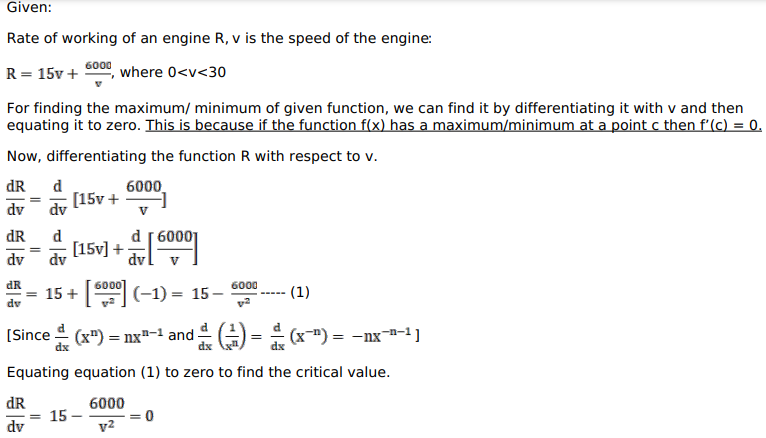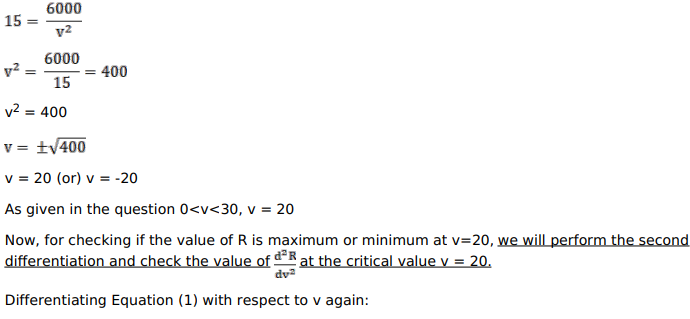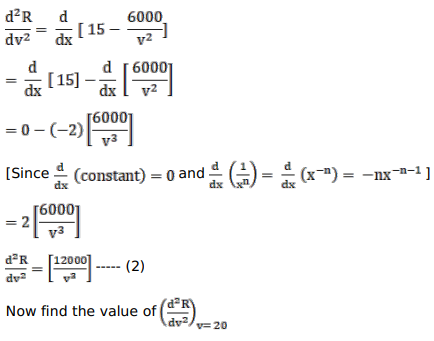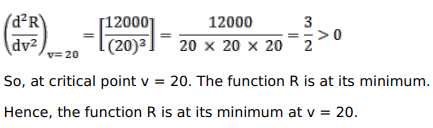# The rate of working of an engine is given by.

Question:

The rate of working of an engine is given by.

$R=15 v+\frac{6000}{v}$, where $0 and$v$is the speed of the engine. Show that$R$is the least when$v=20\$.

Solution: